# Plan of the village

Plan of the municipality in 1:1000 scale has plotted garden with dimensions 25 mm and 28 mm. Determine the area of gardens in ares in reality.

S =  7 a

### Step-by-step explanation: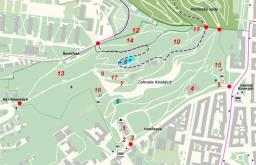Did you find an error or inaccuracy? Feel free to write us. Thank you!Tips to related online calculators
Check out our ratio calculator.
Do you want to convert length units?

#### You need to know the following knowledge to solve this word math problem:

We encourage you to watch this tutorial video on this math problem:

## Related math problems and questions:

• Scale of planOn the plan of the village in the scale of 1: 1000 a rectangular garden is drawn. Its dimensions on the plan are 25mm and 28mm. Determine the area of the garden in ares.
• Map scaleGarden has on plan on a scale of 1: 150 width 22 cm and length 35 cm. What is the real area of the garden?
• Photo egative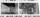Negative dimensions are 36mm and 28mm. What will be the photo size in the 21:4 ratio?
• Map scale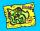What is the scale of the map, if 5.4 km is 72 mm on the map?
• Geometric plan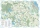At what scale the building plan if one side of the building is 45m long and 12mm long on a plan?
• The scaleThe distance of 15 cm on the map corresponds to a distance of 27 km in reality. What is the scale of the map?
• Two monuments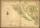The distance between two historical monuments on a map with a scale of 1: 500000 is 48 mm. Find the actual distance between these monuments in km.
• Square garden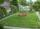On the plan with a scale of 1:1500 is drawn as a square garden with area 81 cm2. How many meters is long garden fence? Determine the actual acreage gardens.
• Map scaleThe rectangular plot has a scale of 1: 10000 area 3 cm2 on the map. What content does this plot have on a 1:5000 scale map?
• Hectares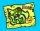Determine the area of the rectangular land (in ha), which has dimensions of 3 cm and 4.5 cm on the plan with a scale of 1: 40,000.
• Scale of the mapDetermine the map's scale, which is the actual distance of 120 km l represented by a segment long 6 cm.
• The sidewalk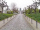The sidewalk is 50 m long. What will be its length on the plot plan in the scale of 1: 200?
• Hřiště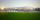Map scale is 1: 5000. The playground is rectangular and on the map has dimensions 10 cm and 5 cm What is area of playground in square meters in reality?
• PlotThe plot on which Mr. Kalous is to build a house has the shape of a rectangle. On a 1: 500 scale, its dimensions are 7cm and 5.5cm. Find out the dimensions of the plot. Calculate the parcel size.
• Map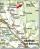Forest has an area of ​​36 ha. How much area is occupied by forest on the map at scale 1:500?
• Small city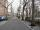The length of the street is 230 m. What will be the length of its image on the plan at a scale of 1: 2500?
• Field on plan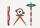The plan has a scale of 1: 2500. Determine dimensions in centimeters of the plan if the field has a length of 310 meters and 182.5 meters.